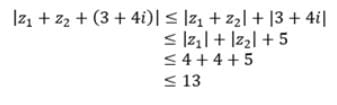# Test: Geometry Of Complex Numbers

## 10 Questions MCQ Test Mathematics For JEE | Test: Geometry Of Complex Numbers

Description
Attempt Test: Geometry Of Complex Numbers | 10 questions in 10 minutes | Mock test for JEE preparation | Free important questions MCQ to study Mathematics For JEE for JEE Exam | Download free PDF with solutions
QUESTION: 1

### For a complex number x + iy, if x > 0 and y < 0 then the number lies in the.

Solution:

When x > 0 & y < 0, the quadrant contains positive X-axis and negative Y-axis
Therefore, the point lies in the fourth quadrant.

QUESTION: 2

### If U = set of all whole numbers less than 12, A = set of all whole numbers less than 10, B = Set of all odd natural numbers less than 10, then what is (A∩B)’?

Solution:

U = {0, 1, 2, 3, 4, 5, 6, 7, 8, 9, 10, 11}
A = {0, 1, 2, 3, 4, 5, 6, 7, 8, 9, 10}
B = {1, 3, 5, 7, 9}
A ∩ B = {1, 3, 5, 7, 9}
(A ∩ B)’ = U - (A ∩ B)
(A ∩ B)’ = {0, 2, 4, 6, 8, 10, 11}

QUESTION: 3

### If |z1| = 4, |z2| = 4, then |z1 + z2 + 3 + 4i| is less than

Solution:QUESTION: 4

Which of the following is a finite set?

Solution:
QUESTION: 5

If P and Q are two sets such that n(P) = 120, n(Q) = 50 and n(P ∪ Q) = 140 then, n(P ∩ Q) is:

Solution:

n(P)=120
n(P∪Q)=140
n(Q)=50
n(P∪Q)=n(P)+n(Q)−n(P∩Q)
140 = 120 + 50 - n(P∩Q)
n(P∩Q) = 30

QUESTION: 6

A point z = P(x,y) lies on the negative direction of y axis, so amp(z) =

Solution:

P(-x,-y) lies on the 3rd or 4th quadrant
So, according to the question, points lies in 3rd quadrant
i.e. 3(π/2)

QUESTION: 7

The argument of the complex number -i

Solution:

z = -i
Comlex number z is of the form x + iy
x = 0, y = -1
arg(z) = π − tan−1|y/x|
⇒ π − tan−1|-1/0|
= π - (π/2)
⇒  π - π/2
⇒ π/2

QUESTION: 8

The modulas of the complex number -1 + √3i is

Solution:

√(-1)2 + (√3)2

= 2

QUESTION: 9

The argument of the complex number -1 – √3

Solution:

z = a + ib
a = -1, b = -√3
(-1, -√3) lies in third quadrant.
Arg(z) = -π + tan-1(b/a)
= - π + tan-1(√3)
= - π + tan-1(tan π/3)
= - π + π/3
= -2π/3

QUESTION: 10

The amplitude of a complex number is called the principal value amplitude if it lies between.

Solution:

x = |z| cos θ and y = |z| sin θ satisfies infinite values of θ and for any infinite values of θ is the value of Arg z. Thus, for any unique value of θ that lies in the interval - π < θ ≤ π and satisfies the above equations x = |z| cos θ and y = |z| sin θ is known as the principal value of Arg z or Amp z and it is denoted as arg z or amp z.

We know that, cos (2nπ + θ) = cos θ and sin (2nπ + θ) = sin θ (where n = 0, ±1, ±2, ±3, .............), then we get,

Amp z = 2nπ + amp z where - π < amp z ≤ πUse Code STAYHOME200 and get INR 200 additional OFF Use Coupon Code